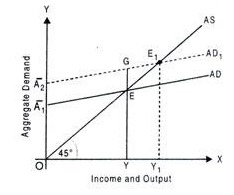# The Short Run Fixed Price Analysis of the Product Market: Concepts etc

The compilation of these Income Determination Notes makes students exam preparation simpler and organised.

## The Short Run Fixed Price Analysis of the Product Market

Do prices remain the same throughout or do they behave differently in different time periods? And if prices are ‘fixed’ and unchanging in the short run, what possible impact could it have on the equilibrium output determination? This lesson on short-run fixed price analysis breaks down the effect of fixed prices in the short run on equilibrium output using AD-AS equations and diagrams. It also examines what happens when the AD curve shifts.

### Fixed Price and the Short-Run

The key terms you must first look at are: ‘short-term’ and ‘fixed price’. In economics, we always distinguish between short-term and long-term. A short period of time, extending for usually less than a year, is called the short -run. A period of time longer than that is termed as long-run. Our variable of interest to carry out a short-run fixed price analysis is naturally, ‘price’.

What is the meaning of Fixed Price and why is it fixed in the Short Run?
By the term fixed price, we mean that price remains constant. They do not change as per demand and supply conditions in the short run. The reason behind this is that there are always chances that aggregate demand and supply do not equalize in an economy. Prices take time to respond to these conditions.

A single firm may not be in a position to influence prices as it might be contributing a very small share to the total market output. Therefore, firms might become individual price takers in the short-run (like the case of perfect competition). For these reasons, in the short run, prices remain fixed. Prices change in the long run.

What is the Economic Impact of Constant prices in the Short Run?
In order for prices to be constant in the short run, we must assume aggregate supply to be infinitely elastic.Why? An infinite elasticity of supply means that suppliers are willing to supply any amount that the consumers will demand at the given fixed price. If supply is not infinitely elastic, excess supply situations might arise and prices will have to change to adjust demand and supply for equilibrium.

Therefore, equilibrium is entirely determined by the aggregate demand. This is known as the effective demand principle. ‘Effective demand’ is the total demand which is met by the corresponding supply level.

Equilibrium Determination under ‘Fixed Price Model’
As stated above, Aggregate Demand (AD) determines the equilibrium in the fixed price model. Let us understand the scenario using a two-sector economy with households and firms.

In this economy, AD equals the sum of Consumption (C) and Investment (I), i.e. AD = C + I

Now, Consumption can be explained with the help of the consumption function. The consumption function depicts the positive relationship between consumption and income (Y). The level of dependence of C on Y is determined by MPC (here, ‘b’). The consumption function must also comprise an ‘autonomous’ component, which is the minimum level of consumption, whatever be the value of income (this is steady even if income is zero). This component is depicted by ‘C0’.

So, C = C0 + b.Y

Here, ‘b’ is the marginal propensity to consume or the increase in consumption per unit increase in income. It is the slope of the consumption function and always lies between 0 and 1.

In the two-sector model, we make the investment to be ‘autonomous’ i.e. it is not affected by the income level, and therefore, we may write: I = I0
We can now write the AD equation from (i) as:

AD = C0 + b.Y + I0
AD = C0 + I0 + b.Y
AD = A + b.Y (clubbing the autonomous components)

Diagrammatic Representation
To depict equilibrium diagrammatically, take income or output on the horizontal axis and aggregate demand on the vertical axis. We take the Aggregate Supply (AS) curve to be a 45º line to show all the points at which aggregate output equals aggregate expenditures. This is necessary to determine equilibrium.

The AD curve is upward sloping and starts from an intercept due to the combined autonomous component (A). The slope of the AD curve is ‘b’ (MPC). Note that equilibrium is the point where Aggregate Supply (AS) equals Aggregate Demand (AD) or where Aggregate Output equals Aggregate Expenditures.We see that the AD curve ‘cuts’ the 45º line at point ‘E’, which is the equilibrium point. Corresponding output level, OY is the equilibrium output. This is the equilibrium situation for fixed prices in the short run.

Example:

Question:
What happens when any of the autonomous components change?